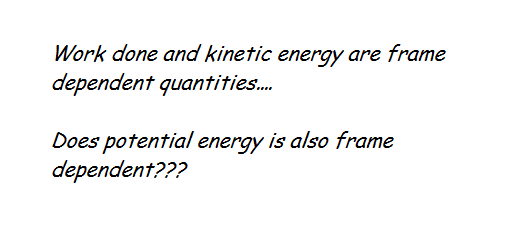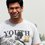# Thought OF The Day_8_Frames and energy!!$\text{Comments Invited!!}$Note by Rohit Gupta
6 years, 1 month ago

This discussion board is a place to discuss our Daily Challenges and the math and science related to those challenges. Explanations are more than just a solution — they should explain the steps and thinking strategies that you used to obtain the solution. Comments should further the discussion of math and science.

When posting on Brilliant:

• Use the emojis to react to an explanation, whether you're congratulating a job well done , or just really confused .
• Ask specific questions about the challenge or the steps in somebody's explanation. Well-posed questions can add a lot to the discussion, but posting "I don't understand!" doesn't help anyone.
• Try to contribute something new to the discussion, whether it is an extension, generalization or other idea related to the challenge.

MarkdownAppears as
*italics* or _italics_ italics
**bold** or __bold__ bold
- bulleted- list
• bulleted
• list
1. numbered2. list
1. numbered
2. list
Note: you must add a full line of space before and after lists for them to show up correctly
paragraph 1paragraph 2

paragraph 1

paragraph 2

[example link](https://brilliant.org)example link
> This is a quote
This is a quote
    # I indented these lines
# 4 spaces, and now they show
# up as a code block.

print "hello world"
# I indented these lines
# 4 spaces, and now they show
# up as a code block.

print "hello world"
MathAppears as
Remember to wrap math in $$ ... $$ or $ ... $ to ensure proper formatting.
2 \times 3 $2 \times 3$
2^{34} $2^{34}$
a_{i-1} $a_{i-1}$
\frac{2}{3} $\frac{2}{3}$
\sqrt{2} $\sqrt{2}$
\sum_{i=1}^3 $\sum_{i=1}^3$
\sin \theta $\sin \theta$
\boxed{123} $\boxed{123}$

Sort by:

well, it depends on the chosen refference point, but for a given refference point , does it vary between frames?

well that depends on the force law that defines it,

but how about this, if we have a electromagnetic situation, surely it depends on frame of observation

magnetic field in one frame is electric field in another, and hence the potential too must vary, as the contribution of electric field too the total field changes

but, i do not think there is any other situation, what is the solution?

- 6 years, 1 month ago

First let us talk about gravitational potential energy..!! If We talk about slow speeds then mass is nearly invariant and so does gravitational force and therefore potential energy is invariant for Newtonian physics. Gravitational potential energy between point masses depends upon their mass and their relative distance. the distance between the masses and mass of the masses will remain same for slow speed and thus all the frames will say the same potential energy if they take the same zero potential energy configuration. But if we talk about relativistic speeds then mass increases length contracts thus gravitational potential energy may vary and so does the kinetic energy..!!

Similarly in different frame electric field and magnetic field are different therefor electrostatic potential energy is frame dependent as well charge is though invariant but electric field is variant of frame of reference..!!

- 6 years, 1 month ago

yes sir, however i have read that newtonian expression doesnt hold for gravity in relativity , so substituting $\gamma m$ for m does not suffice, but yes the potential depends on frame

thanks for this question , it was enlightening

- 6 years, 1 month ago

Yes , potential energy is frame dependent.

- 6 years, 1 month ago

What about gravitational potential energy?? is it also frame dependent?

- 6 years, 1 month ago

Oh, now it makes me really confused!

- 6 years, 1 month ago

Well, i think I derived my thought from the following (may be flawed) thought experiment.

Let v1 be the velocity of the sytem in Frame 1. Let's say that the velocity of the object is v2 in another frame of reference. If v1 is not equal to v2 (and thus kinetic energies are not equal). If total energy is conserved, then Gravitational Potential Energy may be unequal. And therefore that makes it frame dependent. Can you correct me Sir?

Now I am trying to take help of Special Theory of Relativity which I learnt in 7th grade....

- 6 years, 1 month ago

Energy is conserved, not invariant, so you cant say that

(conserved means it is conserved in all inertial frames as long as you stick to one,

invariant means that it may not be conserved but it will not change from frame to frame at any given instant (like mass) ( it is both conserved and invariant in newtonian mechanics)(only invariant in SR))

- 6 years, 1 month ago

Oh, Thanks for correction.

- 6 years, 1 month ago

also, one thing, in SR, some may disagree that mass is invariant

actually it is a matter of definition

some define $m_o$ as rest mass and moving mass as $\gamma m_o$ , for them , mass is not invariant and momentum and energy is given by mv and $mc^2$

whereas i and many others think its better to define a fixed mass $m$ and instead change the momentum and energy expressions as $\gamma m v$ and $\gamma m c^2$

this is more convenient as you dont have to put the word rest every time you say mass

however, another reason is that the mass changes in inelastic collisions as well (heat lost in newtonian collisions builds up as mass in SR) , and hence instead of having to define mass as a quantity that changes in both inelastic collisions and motion

it is simpler to define a fixed mass that only changes in inelastic collisions, and alter the expressions for momentum and energy instead

so for us, mass is an invariant but not necessarily conserved in a collision

- 6 years, 1 month ago

Basically mass and energy are two different forms of one thing and they are inter convertable and in any situation the sum of mass and energy is conserved!!

- 6 years, 1 month ago

I think gravitational potential energy is not frame dependent in normal conditions.

- 6 years, 1 month ago